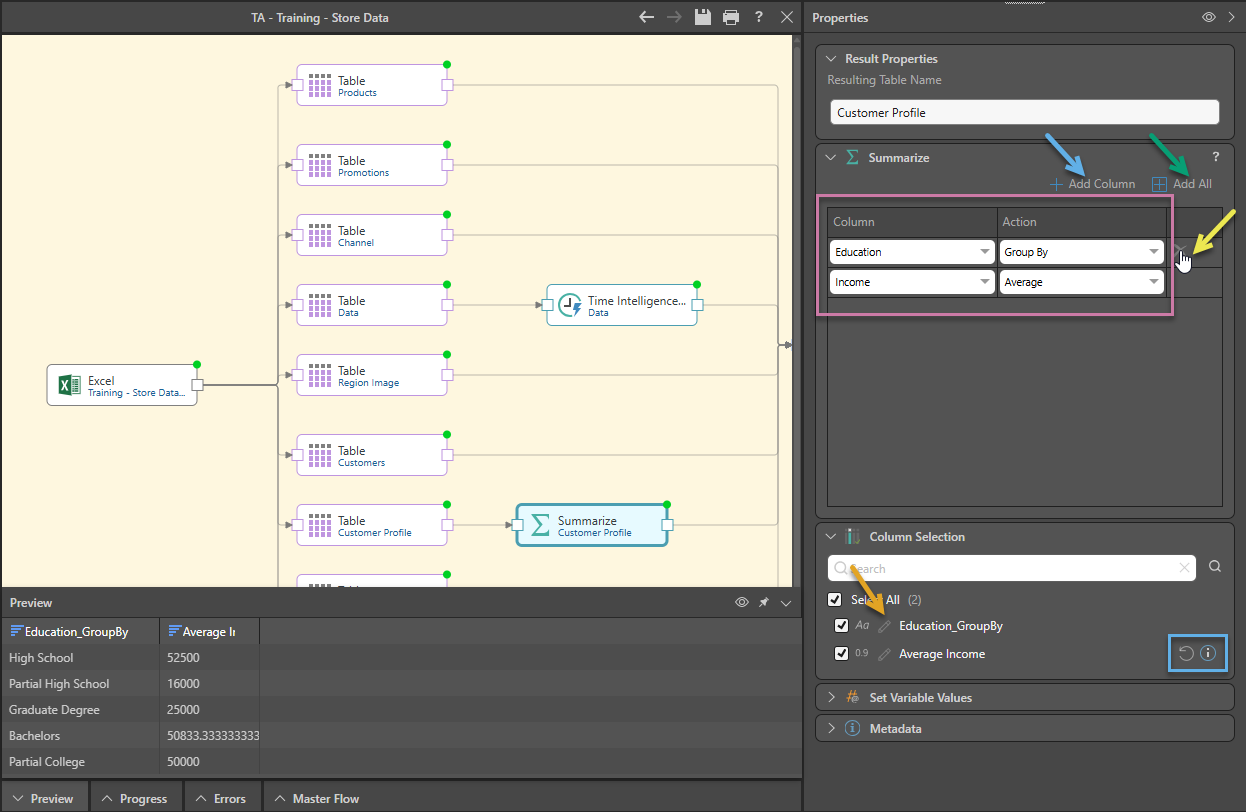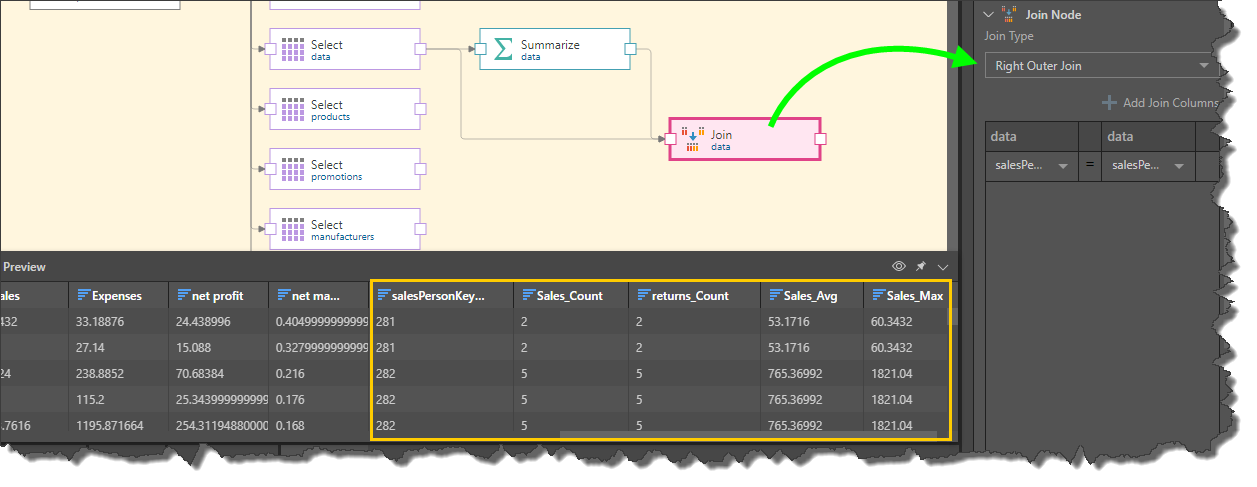# Summarize

The Summarize node is used to construct a new summary table by grouping and aggregating specified columns.

In the example below, the Education column was added to the Summarize node and the Group By function applied. Then the Income column was added and the Average function applied. The resulting table displays the 5 education groups, and the average income for each group.

## How to Configure a Summarize Node

Connect the Summarize node to the Select node representing the relevant table. With the Summarize node selected, go to the Properties panel to set the summarize columns:

1. Click 'Add New Column' (blue arrow below) to add the columns to be summarized.
2. For each column added (red highlight), define the required action (yellow arrow).
3. To remove a column, hover over its listing and click the delete icon (purple arrow).4. To produce a table consisting of the columns from the original table and the Summarize columns, add a Join node to the canvas and connect it to both the table node and the Summarize node.
5. From the Join node's Properties panel, go to the Join Node window; set Right Outer Join as the join type. Next, set the join columns; both columns must exist in both the original table and in the Summarize table.
6. Preview the Join node to see a preview of the table with the Summarize columns.### Summarize Functions

The following functions may be used to generate the Summarize columns:

• Group By: group the summarized columns by this column.
• Count: returns the number of rows in the column.
• Sum: sums the rows in the numeric column.
• Count Non Null: returns the number of rows in the column, excluding nulls.
• Count Null: returns the number of nulls in the column.
• Average: returns the column average for a numeric column.
• Average, ignoring zeros: returns the column average for a numeric column, excluding zero values.
• Min: returns the numeric column's smallest value.
• Max: returns the numeric column's largest value.

## Join the Summarize Node

The Summarize node generates a new table which can then be joined to the original table. This can be done in either Data Flow, using the Join nodes, or in Data Model. The Data Flow is the ETL toolset for Pyramid users; join operations added to the data flow are loaded into the target and stored in the database.

Data Model, on the other hand, is the semantic layer of logic that is used to describe the structure of the data model. Joins configured in Data Model are not added to the database schema, but are used only in the querying process.